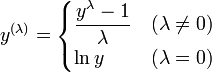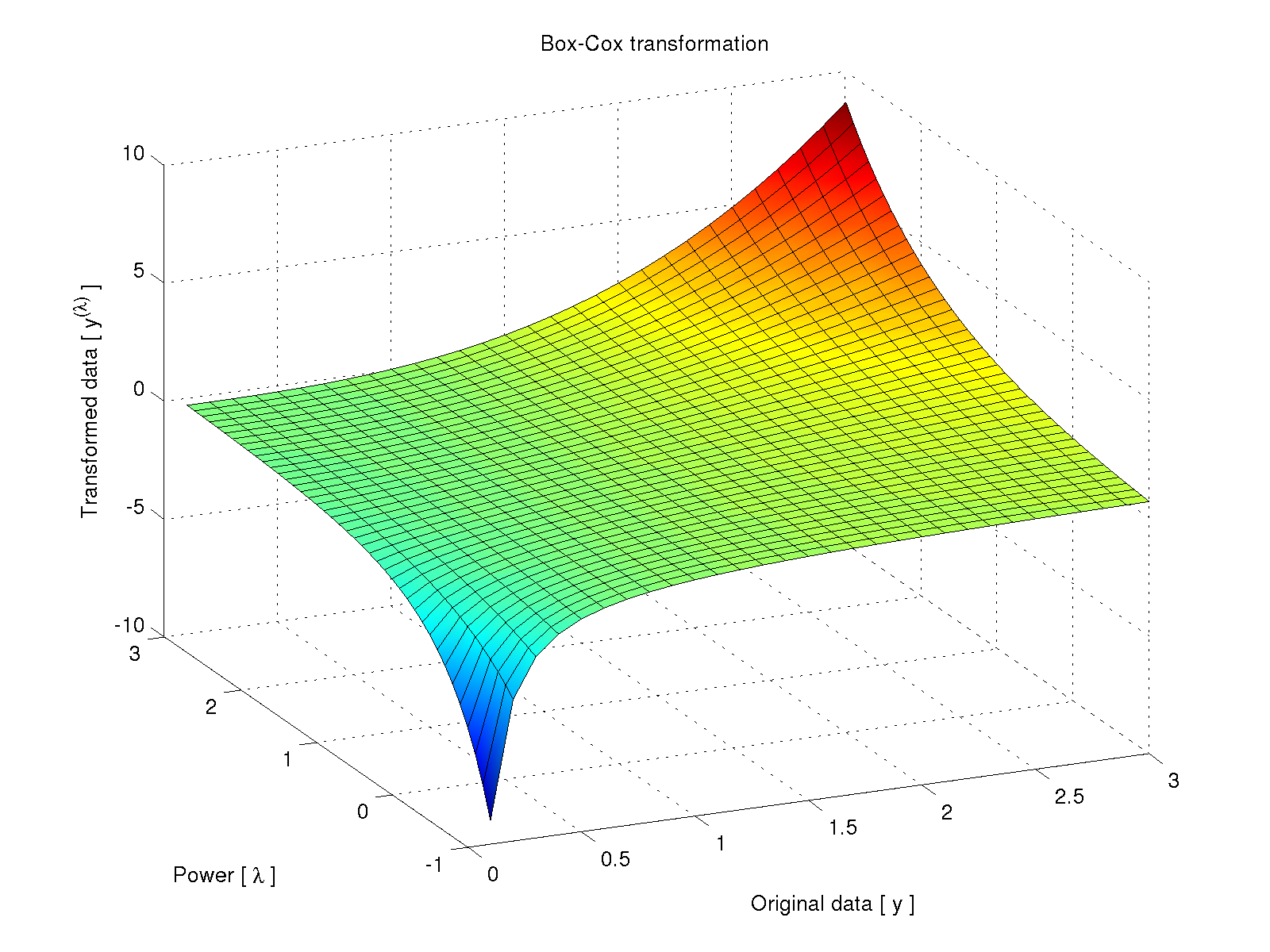# Log-normality and the Box-Cox transformation

The Box-Cox transformation (Box and Cox, 1964) is a way to transform data that ordinarily do not follow to a normal distribution so that it then conforms to it. The transformation is a piecewise function of the power parameter$\lambda$:The function is, given the definition, continuous at the singular point$\lambda = 0$. Plotting the transformation as a function of the original data$y$ and the parameter$\lambda$ produces the following:An interesting aspect of the Box-Cox transformation is that it can be used as a metric to quantify how normal or log-normal certain data are. This has clear applications in biology. Tissue growth that depends on cellular multiplication is exponential, with a constant factor that is often normally distributed, resulting in tissue size that is log-normally distributed (for discussion, see McAlister, 1879; Koch, 1966; Koch, 1969). Measurements of the final tissue size in different individuals, however, may not perfectly conform to the log-normal due to external influences that may hinder tissue growth. Moreover, it is not always possible to measure the final amount of tissue that is the product of a single lineage of self-multiplicative cells. The combination of different cell lineages, each with their own growth rate, as well as external influences, tend to produce a distribution that is more normally (Gaussianly) distributed. A distribution gradient, therefore, may exist between these two extremes of normality and log-normality. Estimating the parameter$\lambda$ allows one to estimate also how closer to normal or log-normal certain measurements are. If$\lambda \approx 0$, the original data tend to be log-normally distributed, whereas if$\lambda \approx +1$, the data can be considered approximately more normally distributed.